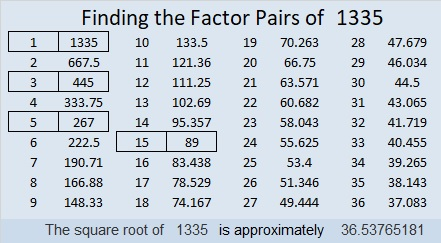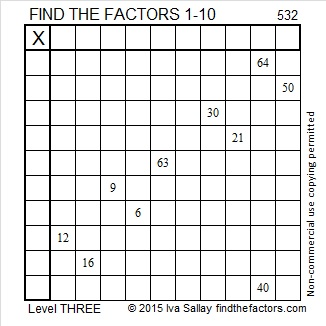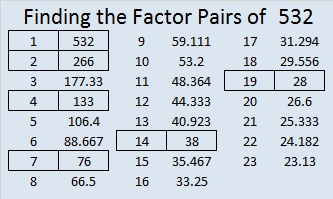# 1335 is a Pentagonal Number

1335 is the 30th Pentagonal Number. Here’s a graphic to help you visualize that fact:Here are some more facts about the number 1335:

• 1335 is a composite number.
• Prime factorization: 1335 = 3 × 5 × 89
• 1335 has no exponents greater than 1 in its prime factorization, so √1335 cannot be simplified.
• The exponents in the prime factorization are 1, 1, and 1. Adding one to each exponent and multiplying we get (1 + 1)(1 + 1)(1 + 1) = 2 × 2 × 2 = 8. Therefore 1335 has exactly 8 factors.
• The factors of 1335 are outlined with their factor pairs in the graphic below.1335 is also the hypotenuse of FOUR Pythagorean triples:
252-1311-1335 which is 3 times (84-437-445)
585-1200-1335 which is 15 times (39-80-89)
609-1188-1335 which is 3 times (203-396-445)
801-1068-1335 which is (3-4-5) times 267

# 1247 Is a Pentagonal Number

Two factors of 1247 make it the 29th pentagonal number. Here’s why:

29(3·29-1)/2 = 29(86)/2 = 29(43) = 1247

Here is an illustration of this pentagonal number featuring a different, but equivalent, formula.  Seeing the pentagonal numbers less than 1247 in the illustration won’t be difficult either.Here are some more facts about the number 1247:

• 1247 is a composite number.
• Prime factorization: 1247 = 29 × 43
• The exponents in the prime factorization are 1 and 1. Adding one to each and multiplying we get (1 + 1)(1 + 1) = 2 × 2 = 4. Therefore 1247 has exactly 4 factors.
• Factors of 1247: 1, 29, 43, 1247
• Factor pairs: 1247 = 1 × 1247 or 29 × 43
• 1247 has no square factors that allow its square root to be simplified. √1247 ≈ 35.312891247 is the sum of consecutive prime numbers two different ways:
It is the sum of the twenty-three prime numbers from 11 to 103.
It is also the sum of seven consecutive primes:
163 + 167 + 173 + 179 + 181 + 191 + 193 = 1247

1247 is the hypotenuse of a Pythagorean triple:
860-903-1247 which is (20-21-29) times 43

# 715 and Level 5

• 715 is a composite number.
• Prime factorization: 715 = 5 x 11 x 13
• The exponents in the prime factorization are 1, 1, and 1. Adding one to each and multiplying we get (1 + 1)(1 + 1)(1 + 1) = 2 x 2 x 2 = 8. Therefore 715 has exactly 8 factors.
• Factors of 715: 1, 5, 11, 13, 55, 65, 143, 715
• Factor pairs: 715 = 1 x 715, 5 x 143, 11 x 65, or 13 x 55
• 715 has no square factors that allow its square root to be simplified. √715 ≈ 26.73948.Can you solve today’s puzzle?Print the puzzles or type the solution on this excel file: 12 Factors 2015-12-14

————————————————————————————

Here are several more facts about the number 715:

715 is the 22nd pentagonal number because 22(3⋅22 – 1)/2 = 715.

715 can be written as the sum of consecutive numbers several ways:

• 357 +358 = 715; that’s 2 consecutive numbers.
• 141 + 142 + 143 + 144 + 145 = 715; that’s 5 consecutive numbers.
• 60 + 61 + 62 + 63 + 64 + 65 + 66 + 67 + 68 + 69 + 70 = 715; that’s 11 consecutive numbers.
• 49 + 50 + 51 + 52 + 53 + 54 + 55 + 56 + 57 + 58 + 59 + 60 + 61 = 715; that’s 13 consecutive numbers.

Since 5 and 13 are two of its factors, 715 is the hypotenuse of four Pythagorean triples.

• 176-693-715
• 275-660-715
• 363-616-715
• 429-572-715

Each of those triples has a greatest common factor. Can you find them all?

715 is also palindrome 1D1 in BASE 21 where D = 13 base 10; note that 1(21²) + 13(21) + 1(1) = 715.

————————————————————————————# 532 and Level 3

532 is the sum of consecutive prime numbers 263 and 269.

532 is also the 19th pentagonal number.Print the puzzles or type the solution on this excel file: 10 Factors 2015-06-22

—————————————————————————————————

• 532 is a composite number.
• Prime factorization: 532 = 2 x 2 x 7 x 19, which can be written 532 = (2^2) x 7 x 19
• The exponents in the prime factorization are 1, 2, and 1. Adding one to each and multiplying we get (2 + 1)(1 + 1)(1 + 1) = 3 x 2 x 2 = 12. Therefore 532 has exactly 12 factors.
• Factors of 532: 1, 2, 4, 7, 14, 19, 28, 38, 76, 133, 266, 532
• Factor pairs: 532 = 1 x 532, 2 x 266, 4 x 133, 7 x 76, 14 x 38, or 19 x 28
• Taking the factor pair with the largest square number factor, we get √532 = (√4)(√133) = 2√133 ≈ 23.065125—————————————————————————————————

A Logical Approach to solve a FIND THE FACTORS puzzle: Find the column or row with two clues and find their common factor. Write the corresponding factors in the factor column (1st column) and factor row (top row).  Because this is a level three puzzle, you have now written a factor at the top of the factor column. Continue to work from the top of the factor column to the bottom, finding factors and filling in the factor column and the factor row one cell at a time as you go.